# Perfect for differentiation!

Classroom teachers can work with a small group of students while the class works with Michelle via video - from any device!

Spelling lessons from Foundation (Prep) right through to Year 6.

#### A lesson on every spelling pattern from the manual!

The 227 ten minute video lessons include 35 lessons for Foundation (Prep) level and 32 lessons for each Year 1 - 6 level. Each student will have access to every lesson from one login.  The teacher can instruct each student as to which lessons to watch, depending on their individual need.  This allows for differentiation as students can work at any year level that their teacher has directed.

These lessons are not organised by year level but by lesson number.  The lesson number matches the page number of that spelling pattern in the manual.

#### Perfect for differentiation!

Teachers can work with a small group of students while the class works with Michelle via video - from any device. Alternatively the lesson can be projected at the front of the classroom.

The video lessons are optional for schools who already use this approach and are accessed by logging into this website.

## For a Whole School Subscription

With access now, right through until the end of 2021!

We'll set up your entire school with one login to use school wide.  All staff and students will have access to all 227 lessons from that one login.

One login - Making life easier!

Payment can be made in 2021 to help with budgeting.

Payment is either by bank transfer later or by credit card at the time of purchase.  If you have a purchase order number, enter it at checkout and if not, just leave it blank.  A tax invoice will be emailed to you automatically after purchase.

# FAQs

What does 'whole school' mean?

If you're a primary school then it means Foundation to Year 6, your current enrolment numbers.  If you are in a F-12 school, then it means your junior school.  If you're in a situation where just some of your school uses SMART Spelling, please email us to explore other pricing options.

Until the end of 2021.

How do we get the weekly spelling sheets for each lesson?

Do I order for 2020 numbers or projected numbers for next year?

Just use 2020 numbers.

As SMART Spelling is used all over Australia, which font have you used?

Comic Sans but students can write their spelling words in whichever font they are used to.

Do we need to be using SMART Spelling at our school?

Yes.  These lessons are for schools that have trained their teachers in this approach.

How do we access the lessons?

The videos are hosted on our website (the videos are not downloadable).  You simply login once to access all of them.

Can we host the videos on our own platform?

No, they are only hosted on our website. This way we can ensure the correct weekly spelling sheets (and rules when needed) are there to download easily.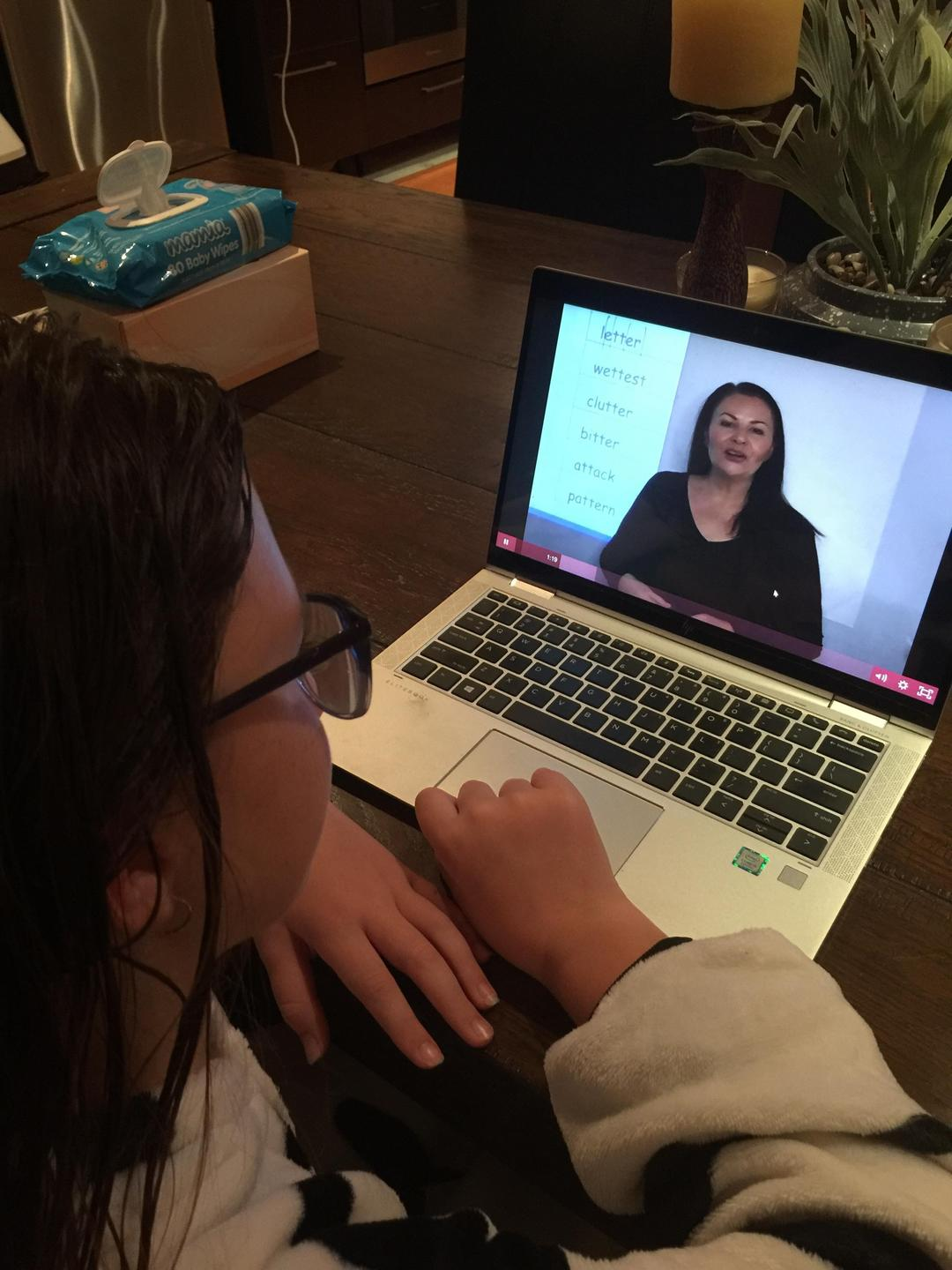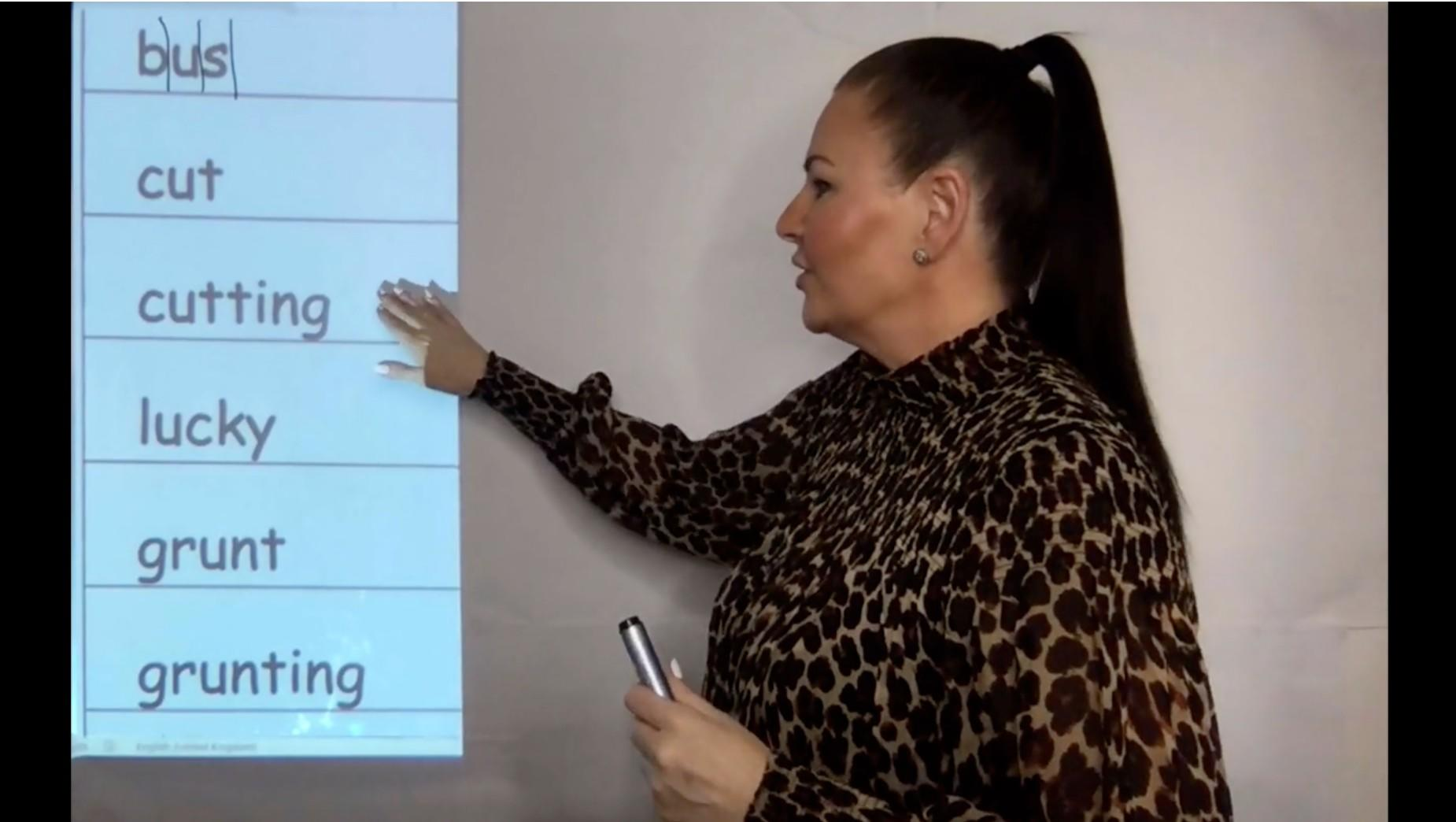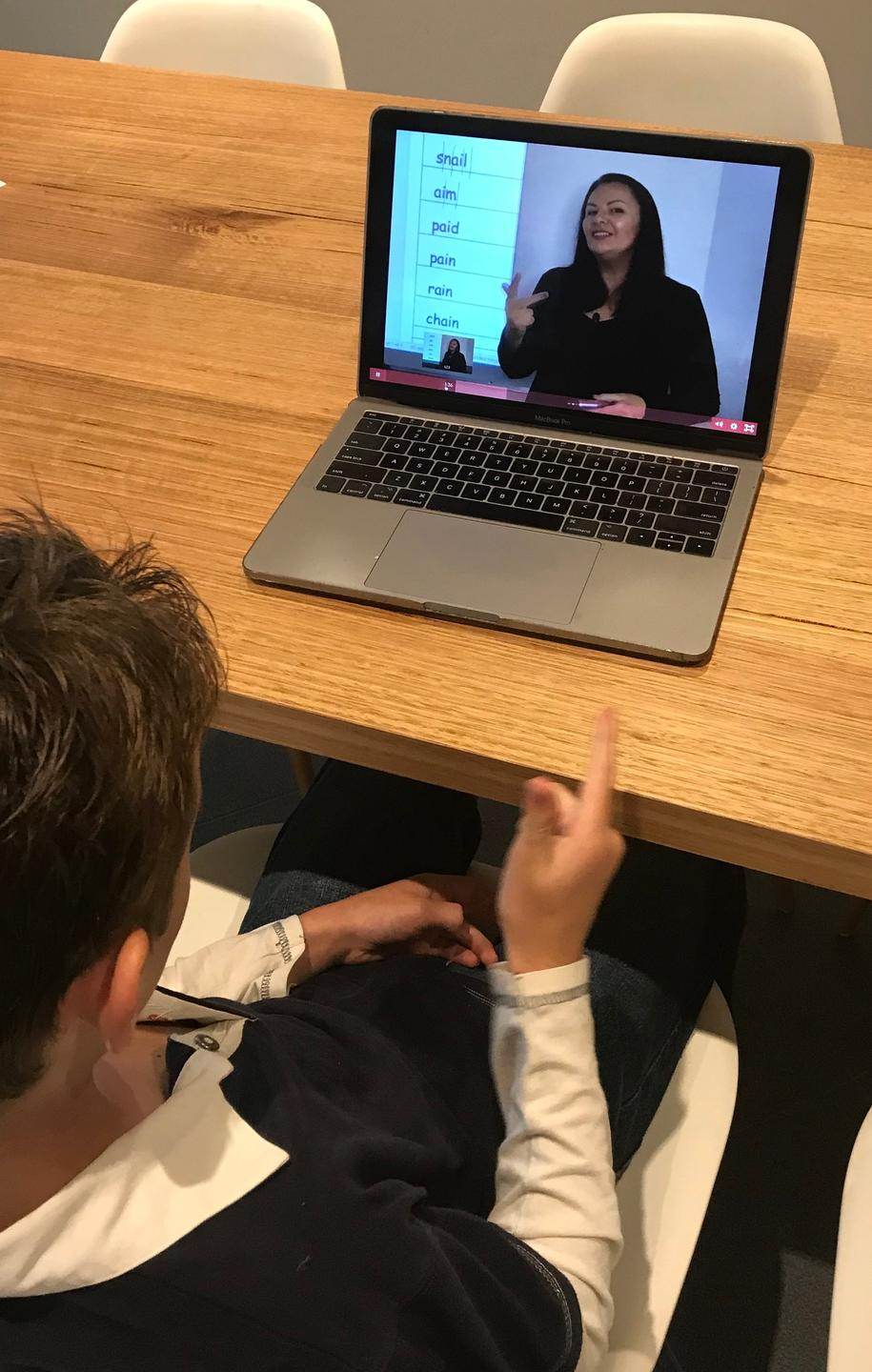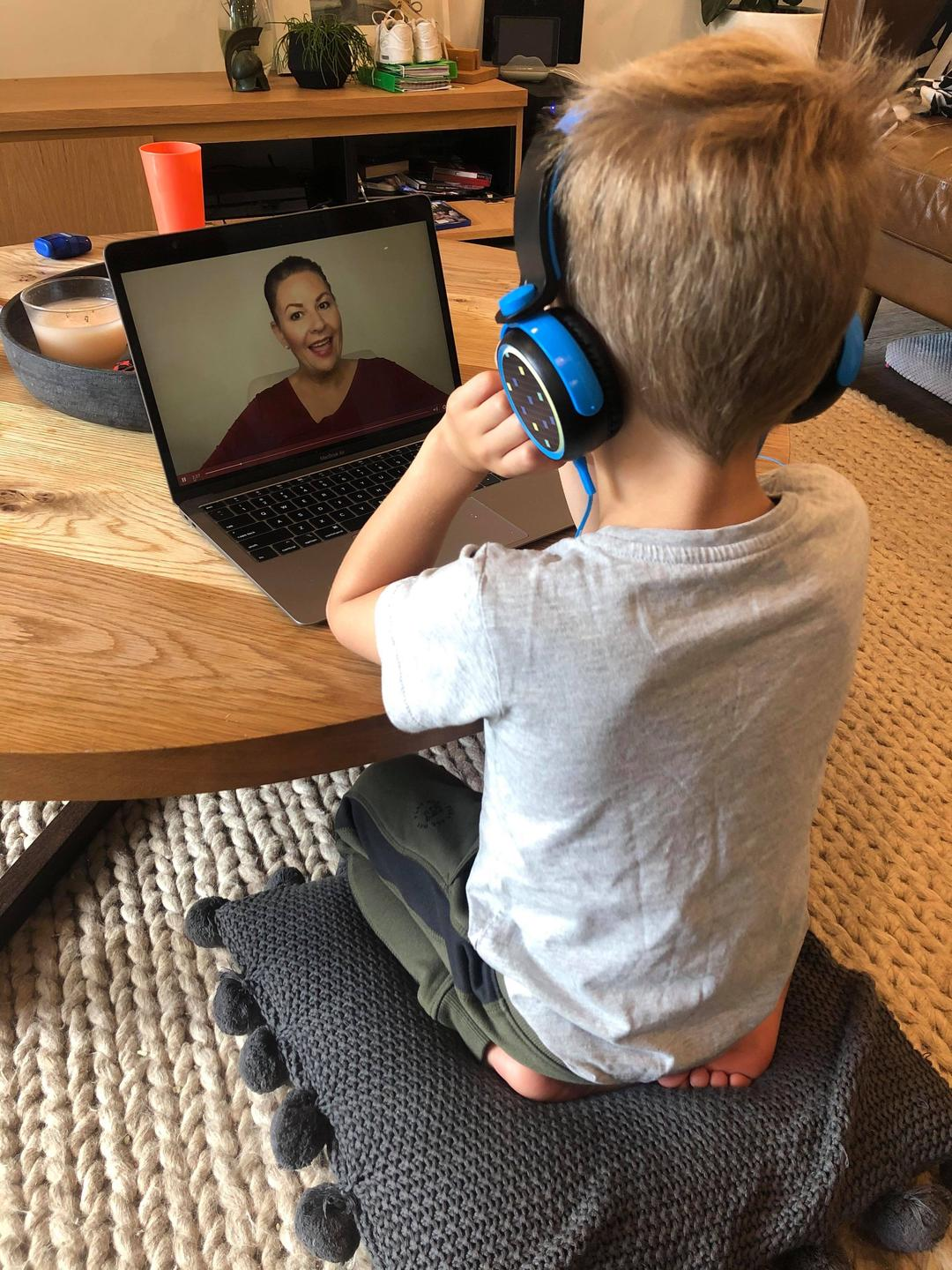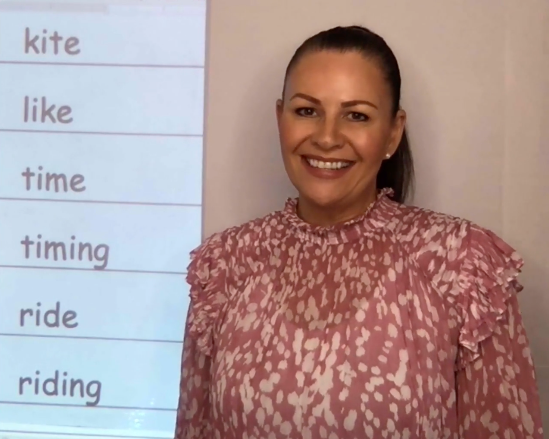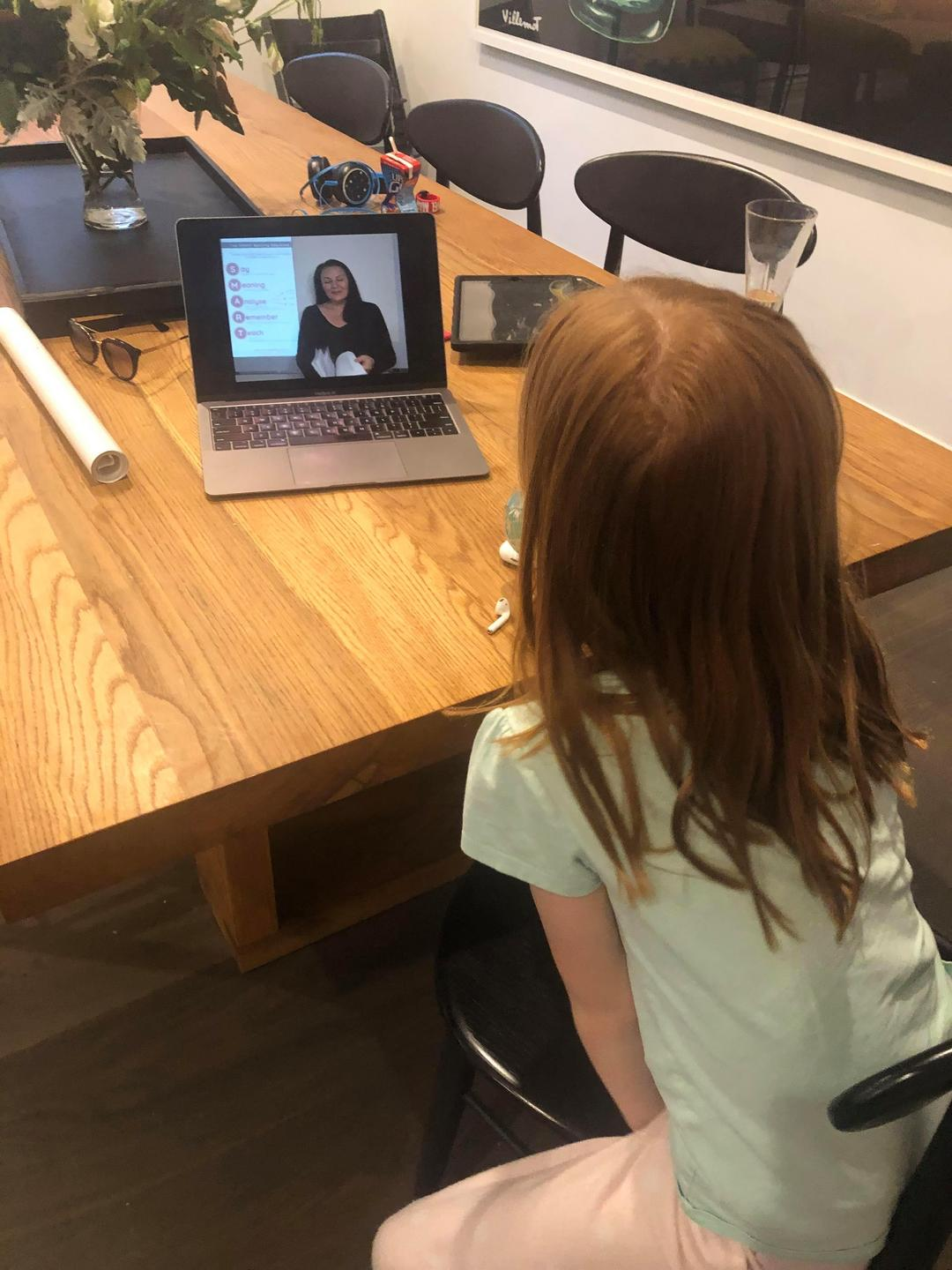## What the lessons cover

Foundation/Prep level:

There are 35 lessons. Each lesson has a 5 minute 'warm up' phonological activity and then a 5 minute explicit lesson on new material. There are some demo lessons here.

Phase 1: cat, ant, tin, tap (and the related common words from the sentences of the week).

Phase 2: sun, net, leg, bed (and the related common words from the sentences of the week).

Phase 3: dog, jam, hand, frog (and the related common words from the sentences of the week).

Phase 4: zip, yes, wax, vet (and the related common words from the sentences of the week).

Phase 5: car, king, tray, shark (and the related common words from the sentences of the week).

Phase 6: duck, beach, teacher, feather (and the related common words from the sentences of the week).

Phase 7: fork, thumb, wheel, queen (and the related common words from the sentences of the week).

Phase 8: swan, watch, hair, light (and the related common words from the sentences of the week).

Phase 9: tape, kite, note (and the related common words from the sentences of the week).

#### For Years 1 - 6 the lesson number matches the page number in the manual.

Year 1
Lesson 58 The graph /a/ making the sound "a" as in cat

Lesson 59 The graph /y/ making the sound “I” as in fly.

Lesson 60 The graph /o/ making the sound "o" as in frog

Lesson 61 The digraph /ar/ making the sound "ar" as in car.

Lesson 62 The graph /e/ making the sound "e" like in bed.

Lesson 63 The digraph /ck/ making the sound 'k" usually at the end of words.

Lesson 64 The graph /i/ making the sound "i" as in tin.

Lesson 65 the graph /k/ making the sound "k" as in kitten.

Lesson 66 The digraph /oo/ making the sound "oo" as in book.

Lesson 67 The graph /u/ making the sound "u" as in bus and the prefix un- meaning 'not'.

Lesson 68 The digraph /ng/ making the sound "ng" as in king.

Lesson 69 The digraph /sh/ making the sound “sh” as in shark.

Lesson 70 The digraph /ee/ making the sound “E” as in tree.

Lesson 71 The graph /a/ making the sound "o" as in swan.

Lesson 72 The split digraph /a- -e/ making the sound “A” and the yellow spelling rule.

Lesson 73 The split digraph o - e making the sound "O" as in note and the yellow spelling rule.

Lesson 74 The split digraph /i - e/ making the sound "I" as in kite and the yellow spelling rule.

Lesson 75 The digraph /ze/ making the sound "z" as in sneeze and the yellow spelling rule.

Lesson 76 The digraph /th/ making the sound "th" as in thumb.

Lesson 77 The digraph /ow/ making the sound “ou” as in cow.

Lesson 78 The digraph /ay/ making the sound "A" as in tray.

Lesson 79 The digraph /wh/ making the sound "w" as in wheel.

Lesson 80 The digraph /oo/ making the sound “oo” as in moon.

Lesson 81 The digraph /ir/ making the sound "er" as in shirt.

Lesson 82 The trigraph /tch/ making the sound “ch” as in watch.

Lesson 83 The digraph /or/ making the sound "or" as in fork.

Lesson 84 The digraph /th/ making the sound “th” as in feather.

Lesson 85 The digraph /oa/ making the sound “O” as in boat.  Usually in the middle of base words.

Lesson 86 The digraph /ch/ making the sound ”ch” as in chair.

Lesson 87 The graph /a/ making the sound “or” as in ball.

Lesson 88 The digraph /ss/ making the sound "s" as in dress.

Lesson 89 The digraph /oy/ making the sound “oy” as in toy.

## Year 2

Lesson 92 The digraph /ai/ making the sound “A” as in snail.

Lesson 93 The digraph /ur/ making the sound “er” as in fur.

Lesson 94 The digraph /ow/ making the sound “O” as in snow

Lesson 95 The graph /y/ making the sound “E” as in pony.

Lesson 96 The trigraph /dge/ making the sound “j” as in bridge.

Lesson 97 The trigraph /air/ making the sound "air" as in hair.

Lesson 98 The graph /a/ making the sound "ar" as in class.

Lesson 99 The digraph /ea/ making the sound “e” as in bread.

Lesson 100 The digraph /ey/ making the sound "E" as in key.

Lesson 101 The digraph /ea/ making the sound “E” as in beach.

Lesson 102 The digraph /er/ making the sound "eh" as in teacher.

Lesson 103 The digraph /oi/ making the sound "oy" as in coin.

Lesson 104 The graph /u/ making the sound "oo" as in bull.

Lesson 105 The digraph /or/ making the sound "or" as in fork.

Lesson 106 The digraph /ou/ making the sound "ou" as in house.

Lesson 107 The graph /o/ making the sound "u" as in glove.

Lesson 108 The trigraph /igh/ making the sound "I" as in light.

Lesson 109 Plural Rule 2 - Adding es.

Lesson 110 The graph /a/ making the sound "a" and the blue spelling rule.

Lesson 111 The graph /e/ making the sound "e" and revision of the blue spelling rule

Lesson 112 The graph /i/ making the sound "i" as in tin and the blue spelling rule.

Lesson 113 The graph /o/ making the sound "o" as in frog and the blue spelling rule.

Lesson 114 The graph /u/ making the sound "u" as in bus and the blue spelling rule

Lesson 115 The prefix /re-/ meaning repeating or backwards.

Lesson 116 The digraph /mb/ as in lamb and the graph /m/ as in mouse, both making the sound "m".

Lesson 117 The digraph /wr/ making the sound "r" as in wrist.

Lesson 118 The digraph /kn/ making the sound "n" as in knee.

Lesson 119 The digraph /ay/ making the sound “A” as in tray.

Lesson 120 The graph /f/ making the sound "f" as in fish.

Lesson 121 The digraph /ll/ making the sound "l" as in bell.

Lesson 122 The split digraph /i- -e/ making the sound “I” as in kite.

Lesson 123 The graph /s/ making the sound “s” as in sun.

## Year 3

Lesson 126 The digraph /er/ making the sound “er” as in fern.

Lesson 127 The digraph /ge/ making the sound “j” as in cage.

Lesson 128 The graph /y/ making the sound "E" and the pink spelling rule.

Lesson 129 The digraph /or/ making the sound "er" as in worm.

Lesson 130 Lesson 130 Words that end  in /l e/  as in table.

Lesson 131 The graph /y/ making the sound "i" as in pyramid.

Lesson 132 The trigraph /are/ making the sound “air” and the yellow spelling rule.

Lesson 133 The digraph /ho/ as in honest and the graph /o/ making the sound "o".

Lesson 134 The trigraph /ear/ making the sound "ear" as in ear.

Lesson 135 The graph /c/ making the sound “s” as in city.

Lesson 136 The prefix inter- meaning 'between'.

Lesson 137 Contractions.

Lesson 138 The digraph /se/ making the sound "s" as in horse.

Lesson 139 The digraph /oi/ making the sound "oy" as in coin.

Lesson 140 The digraph /oy/ making the sound “oy” as in toy.

Lesson 141 The graph /q/ making the sound "k" as in queen

Lesson 142 The graph /a/ making the sound “A” as in baby.

Lesson 143 The graph /a/ and the digraph /al/ both making the sound "ar".

Lesson 144 The graph /g/ making the sound "j" as in giant and revision of the sound "eh".

Lesson 145 The graph /s/ making the sound "zh" as in treasure and revision of the yellow spelling rule.

Lesson 146 The digraph /ti/ making the sound “sh” as in station.

Lesson 147 The graph /u/ making the sound "w" as in quilt.

Lesson 148 The digraph /se/ making the sound "z" as in cheese.

Lesson 149 The digraph /aw/ making the sound “or” as in saw.

Lesson 150 The trigraph /our/ making the sound "eh" as in colour.

Lesson 151 The quadraph /eigh/ and the split digraph a - e both making the sound "A".

Lesson 152 Plural rule 5.  Words ending in y.

Lesson 153 Plural rule 3.  Words ending in f or fe.

Lesson 154 Plurals ending in o.

Lesson 155 The consonant suffix -ful.

Lesson 156 The consonant suffix -less.

Lesson 157 The graph /o/ making the sound “u” as in glove.

## Year 4

Lesson 160 The quadgraph /augh/ and the digraph /au/ making the sound "or".

Lesson 161 The graph /t/ making the sound "ch" as in nature.

Lesson 162 The digraph /re/ making the sound “eh” as in centre.

Lesson 163 The digraph /tt/ making the sound “t” as in letter.

Lesson 164 The digraph /gg/ making the sound "g" and revision of the blue spelling rule.

Lesson 165 The graph /y/ making the sound “i” as in pyramid.

Lesson 166 The digraph /wr/ making the sound “r” as in wrist.

Lesson 167 The trigraph /que/ and the graph /q/ both making the sound "k".

Lesson 168 The digraph /ou/ making the sound “eh” as in famous.

Lesson 169 The digraph /ch/ making the sound "sh" as in chef

Lesson 170 The digraph /ai/ making the sound "A" as in snail.

Lesson 171 The consonant suffix -ment

Lesson 172 The consonant suffix -ly and revision of the pink spelling rule

Lesson 173 The digraph /ce/ making the sound “s” as in ice.

Lesson 174 The digraph /ie/ making the sound "E" as in chief.

Lesson 175 The trigraph /ure/ making the sound “eh” as in measure and revision of the yellow spelling rule.

Lesson 176 The consonant suffix ‘-ness’ and revision of the pink spelling rule.

Lesson 177 The graph /v/ making the sound “v” as in voice.

Lesson 178 The graph /y/ as in yawn and the graph /i/ and as in onion, both making the sound "y".

Lesson 179 The digraph /or/ making the sound "eh" as in doctor.

Lesson 180 The digraph /ui/ as in fruit and the digraph /ew/ and as in screw, both making the sound "oo".

Lesson 181 The digraph /ci/ making the sound "sh" as in special.

Lesson 182 Common errors of r/rr/wr.

Lesson 183 Common errors of n/nn/kn.

Lesson 184 Common errors of m/mm/mb.

Lesson 185 The graph /e/ making the sound "eh" as in garden.

Lesson 186 The prefix ‘dis’ meaning away or negative.

Lesson 187 The graph /l/ and the digraph /ll/.

Lesson 188 The letter /u/ making two sounds "y" and "oo" as in use.

Lesson 189 The graph /a/ making the sound “a” as in ant.

Lesson 190 The prefix /pro-/ and the prefix /anti-/.

Lesson 191 The graph /p/ making the sound "p" as in panda.

## Year 5

Lesson 194 The digraph /ea/ making the sound “e” as in beach.

Lesson 195 The graph /c/ making the sound "k" as in cat.

Lesson 196 The digraph /ou/ making the sound “u” as in young.

Lesson 197 The graph /c/ making the sound "s" as in city.

Lesson 198 The digraph /gh/ and the graph /g/ making the sound "g"

Lesson 199 The digraph /si/ making the sound "zh" as in division.

Lesson 200 The digraph /cc/ making the sound “k” as in soccer.

Lesson 201 The digraph /ph/ making the sound “f” as in dolphin.

Lesson 202 The prefix mis- meaning mistaken or wrong.

Lesson 203 The prefix pre- and the prefix post-

Lesson 204 The digraph /ti/ making the sound “sh” as in station.

Lesson 205 The digraph /gu/ making the sound "g" as in guitar.

Lesson 206 The digraph /st/ making the sound “s” as in castle.

Lesson 207 The split digraph /a- - e/ making the sound "A" as in tape.

Lesson 208 The graph /r/ making the sound "r" as in rain.

Lesson 209 The digraph /ch/ making the sound “k” as in school.

Lesson 210 The graph /i/ making the sound "E" as in ski.

Lesson 211 Plural rule 7 - Use a different word.

Lesson 212 vowel suffix -able and exceptions to the yellow spelling rule.

Lesson 213 The digraph /au/ making the sound "or" as in sauce.

Lesson 214 The digraph /pp/ making the sound ‘p” as in puppy.

Lesson 215 The digraph /ss/ making the sound “s” as in dress.

Lesson 216 The digraph /or/ making the sound “or” as in fork.

Lesson 217 Revision of plurals.

Lesson 218 Homophones.

Lesson 219 The digraph /ar/ and the quadgraph /ough/ making the sound "or".

Lesson 220 Past tense and revision of the blue and pink spelling rules.

Lesson 221 The graph /y/ making the sound "E" as in pony and the pink spelling rule.

Lesson 222 The prefixes uni, bi and tri.

Lesson 223 The graph /a/ making the sound "o" as in swan.

Lesson 224 The graph /e/ making the sound "E" and revision of the sound "eh".

Lesson 225 The graph /a/ making the sound "eh" as in zebra.

## Year 6

Lesson 228 The prefix re- meaning backwards or again.

Lesson 229 The digraph /ch/ making the sound “k” as in school.

Lesson 230 The digraph /ve/ making the sound “v” as in sleeve. Often at the end of words.

Lesson 231 The digraph /or/ making the sound "eh" as in doctor.

Lesson 232 Revision of the 4 SMART Spelling Rules

Lesson 233 The digraph /ei/ making the sound "E" as in receipt

Lesson 234 The prefix pro- and anti-

Lesson 235 The prefix ‘semi-’ meaning ‘partially’.

Lesson 236 The prefix trans- meaning 'across' or 'through'.

Lesson 237 The prefix hyper- meaning ‘over’ and the prefix hypo- meaning ‘under’.

Lesson 238 The vowel suffix -ism that means a 'system' or 'theory/way of thinking'.

Lesson 239 The vowel suffix -ology which means ‘the study of’.

Lesson 240 The suffix -ist which means ‘job’.

Lesson 241 The digraph /ci/ making the sound “sh” as in special.

Lesson 242 The digraph /ps/ and the graph /s/ making the sound "s".

Lesson 243 The digraph /ai/ and /et/ both making the sound "A"

Lesson 244 The digraph /si/ making the sound "zh" as in division.

Lesson 245 The graph /q/ as in queen and the digraph /cq/ saying "k"

Lesson 246 The vowel suffix -ible

Lesson 247 The digraph /sc/ making the sound “s” as in scissors.

Lesson 248 The prefix micro- and the prefix macro-.

Lesson 249 The prefix un- meaning ‘not or opposite’.

Lesson 250 Revision of homophones.

Lesson 251 The graph /c/ making the sound "s" as in city.

Lesson 252 The prefix inter- meaning 'between'.

Lesson 253 Plurals revision.

Lesson 254 The graph /y/ making the sound “i” as in pyramid.

Lesson 255 The digraph /ou/ making the sound "eh" as in famous.

Lesson 256 The digraph /gn/ making the sound “n” as in sign.

Lesson 257 The trigraph /ssi/ making the sound "sh" as in discussion.

Lesson 258 The digraph /ou/ making the sound “ou” as in house.

Lesson 259 The graph /t/  as in nature.